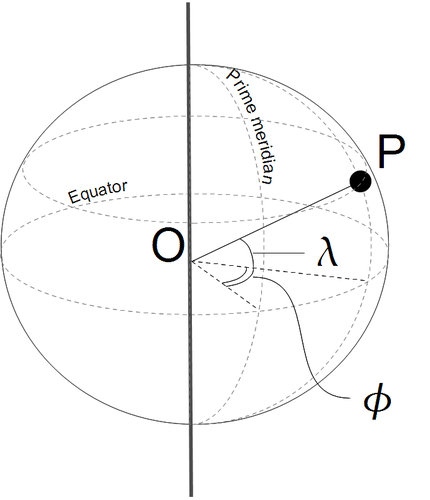10.1: Latitude and Longitude

•• Contributed by CK12
• CK12

Any location on Earth is described by two numbers — its latitude and its longitude. If a pilot or a ship's captain wants to specify position on a map, these are the “coordinates” they would use.

Actually, these are two angles, measured in degrees, “minutes of arc” and “seconds of arc.” These are denoted by symbols e.g. 35 43' 9" means an angle of 35 degrees, 43 minutes and 9 seconds (do not confuse this with the notation (',") for feet and inches!). A degree contains 60 minutes of arc and a minute contains 60 seconds of arc — and you may omit the words “of arc” where the context makes it absolutely clear that these are not units of time.

Calculations often represent angles by small letters of the Greek alphabet, and that way latitude will be represented by $$\lambda$$ (lambda, Greek L), and longitude by $$\phi$$ (phi, Greek F). Here is how they are defined.

Charts used in ocean navigation often use the OPPOSITE notation — $$\lambda$$ for LONGITUDE and $$\phi$$ for LATITUDE. The convention followed here resembles the one used by mathematicians in 3 dimensions for spherical polar coordinates (http://www.phy6.org/stargaze/Scelcoor.htm#q65).Figure $$\PageIndex{1}$$: An illustration of longitude and latitude.